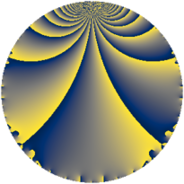# Properties

 Label 5520.2.a.bkLevel $5520$ Weight $2$ Character orbit 5520.a Self dual yes Analytic conductor $44.077$ Analytic rank $1$ Dimension $2$ CM no Inner twists $1$

# Learn more about

## Newspace parameters

 Level: $$N$$ $$=$$ $$5520 = 2^{4} \cdot 3 \cdot 5 \cdot 23$$ Weight: $$k$$ $$=$$ $$2$$ Character orbit: $$[\chi]$$ $$=$$ 5520.a (trivial)

## Newform invariants

 Self dual: yes Analytic conductor: $$44.0774219157$$ Analytic rank: $$1$$ Dimension: $$2$$ Coefficient field: $$\Q(\sqrt{2})$$ Defining polynomial: $$x^{2} - 2$$ Coefficient ring: $$\Z[a_1, \ldots, a_{13}]$$ Coefficient ring index: $$1$$ Twist minimal: no (minimal twist has level 2760) Fricke sign: $$1$$ Sato-Tate group: $\mathrm{SU}(2)$

## $q$-expansion

Coefficients of the $$q$$-expansion are expressed in terms of $$\beta = \sqrt{2}$$. We also show the integral $$q$$-expansion of the trace form.

 $$f(q)$$ $$=$$ $$q - q^{3} + q^{5} + q^{7} + q^{9} +O(q^{10})$$ $$q - q^{3} + q^{5} + q^{7} + q^{9} + ( 2 + 3 \beta ) q^{11} + ( -4 + \beta ) q^{13} - q^{15} + ( -3 + \beta ) q^{17} -5 \beta q^{19} - q^{21} + q^{23} + q^{25} - q^{27} + ( -3 - 3 \beta ) q^{29} + ( 5 - 4 \beta ) q^{31} + ( -2 - 3 \beta ) q^{33} + q^{35} -3 q^{37} + ( 4 - \beta ) q^{39} + ( -3 - \beta ) q^{41} -2 q^{43} + q^{45} + ( -4 + \beta ) q^{47} -6 q^{49} + ( 3 - \beta ) q^{51} + ( -5 + \beta ) q^{53} + ( 2 + 3 \beta ) q^{55} + 5 \beta q^{57} + ( 5 - 5 \beta ) q^{59} + ( -6 - 5 \beta ) q^{61} + q^{63} + ( -4 + \beta ) q^{65} + ( -5 + 4 \beta ) q^{67} - q^{69} + ( 5 + 5 \beta ) q^{71} -11 \beta q^{73} - q^{75} + ( 2 + 3 \beta ) q^{77} + 4 \beta q^{79} + q^{81} + ( -9 + 5 \beta ) q^{83} + ( -3 + \beta ) q^{85} + ( 3 + 3 \beta ) q^{87} + ( -8 + 2 \beta ) q^{89} + ( -4 + \beta ) q^{91} + ( -5 + 4 \beta ) q^{93} -5 \beta q^{95} + ( -4 - 2 \beta ) q^{97} + ( 2 + 3 \beta ) q^{99} +O(q^{100})$$ $$\operatorname{Tr}(f)(q)$$ $$=$$ $$2q - 2q^{3} + 2q^{5} + 2q^{7} + 2q^{9} + O(q^{10})$$ $$2q - 2q^{3} + 2q^{5} + 2q^{7} + 2q^{9} + 4q^{11} - 8q^{13} - 2q^{15} - 6q^{17} - 2q^{21} + 2q^{23} + 2q^{25} - 2q^{27} - 6q^{29} + 10q^{31} - 4q^{33} + 2q^{35} - 6q^{37} + 8q^{39} - 6q^{41} - 4q^{43} + 2q^{45} - 8q^{47} - 12q^{49} + 6q^{51} - 10q^{53} + 4q^{55} + 10q^{59} - 12q^{61} + 2q^{63} - 8q^{65} - 10q^{67} - 2q^{69} + 10q^{71} - 2q^{75} + 4q^{77} + 2q^{81} - 18q^{83} - 6q^{85} + 6q^{87} - 16q^{89} - 8q^{91} - 10q^{93} - 8q^{97} + 4q^{99} + O(q^{100})$$

## Embeddings

For each embedding $$\iota_m$$ of the coefficient field, the values $$\iota_m(a_n)$$ are shown below.

For more information on an embedded modular form you can click on its label.

Label $$\iota_m(\nu)$$ $$a_{2}$$ $$a_{3}$$ $$a_{4}$$ $$a_{5}$$ $$a_{6}$$ $$a_{7}$$ $$a_{8}$$ $$a_{9}$$ $$a_{10}$$
1.1
 −1.41421 1.41421
0 −1.00000 0 1.00000 0 1.00000 0 1.00000 0
1.2 0 −1.00000 0 1.00000 0 1.00000 0 1.00000 0
 $$n$$: e.g. 2-40 or 990-1000 Significant digits: Format: Complex embeddings Normalized embeddings Satake parameters Satake angles

## Atkin-Lehner signs

$$p$$ Sign
$$2$$ $$1$$
$$3$$ $$1$$
$$5$$ $$-1$$
$$23$$ $$-1$$

## Inner twists

This newform does not admit any (nontrivial) inner twists.

## Twists

By twisting character orbit
Char Parity Ord Mult Type Twist Min Dim
1.a even 1 1 trivial 5520.2.a.bk 2
4.b odd 2 1 2760.2.a.q 2
12.b even 2 1 8280.2.a.y 2

By twisted newform orbit
Twist Min Dim Char Parity Ord Mult Type
2760.2.a.q 2 4.b odd 2 1
5520.2.a.bk 2 1.a even 1 1 trivial
8280.2.a.y 2 12.b even 2 1

## Hecke kernels

This newform subspace can be constructed as the intersection of the kernels of the following linear operators acting on $$S_{2}^{\mathrm{new}}(\Gamma_0(5520))$$:

 $$T_{7} - 1$$ $$T_{11}^{2} - 4 T_{11} - 14$$ $$T_{13}^{2} + 8 T_{13} + 14$$ $$T_{17}^{2} + 6 T_{17} + 7$$ $$T_{19}^{2} - 50$$

## Hecke characteristic polynomials

$p$ $F_p(T)$
$2$ $$T^{2}$$
$3$ $$( 1 + T )^{2}$$
$5$ $$( -1 + T )^{2}$$
$7$ $$( -1 + T )^{2}$$
$11$ $$-14 - 4 T + T^{2}$$
$13$ $$14 + 8 T + T^{2}$$
$17$ $$7 + 6 T + T^{2}$$
$19$ $$-50 + T^{2}$$
$23$ $$( -1 + T )^{2}$$
$29$ $$-9 + 6 T + T^{2}$$
$31$ $$-7 - 10 T + T^{2}$$
$37$ $$( 3 + T )^{2}$$
$41$ $$7 + 6 T + T^{2}$$
$43$ $$( 2 + T )^{2}$$
$47$ $$14 + 8 T + T^{2}$$
$53$ $$23 + 10 T + T^{2}$$
$59$ $$-25 - 10 T + T^{2}$$
$61$ $$-14 + 12 T + T^{2}$$
$67$ $$-7 + 10 T + T^{2}$$
$71$ $$-25 - 10 T + T^{2}$$
$73$ $$-242 + T^{2}$$
$79$ $$-32 + T^{2}$$
$83$ $$31 + 18 T + T^{2}$$
$89$ $$56 + 16 T + T^{2}$$
$97$ $$8 + 8 T + T^{2}$$
show more
show less